Ministério da Ciência, Tecnologia, Inovações e Comunicações CBPFIndex CENTRO BRASILEIRO DE PESQUISAS FÍSICAS11/12/2023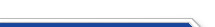Usuário: Senha: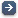Crie sua conta aqui · Busca rápida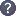Procurar por: Publicações Projetos EventosIr para a busca avançada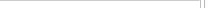Notas de Física
 ${\mathbb Z}_2\times {\mathbb Z}_2$-graded parastatistics in multiparticle quantum Hamiltonians Data do cadastro: 04/09/2020 Publicação/Divulgação: 04/09/2020 Resp. pelo cadastro: Status atual: Publicado Ano da publicação: 2020 Descrição: The recent surge of interest in ${\mathbb Z}_2\times {\mathbb Z}_2$-graded invariant mechanics poses the challenge of understanding the physical consequences of a ${\mathbb Z}_2\times{\mathbb Z}_2$-graded symmetry. \par In this paper it is shown that non-trivial physics can be detected in the multiparticle sector of a theory, being induced by the ${\mathbb Z}_2\times{\mathbb Z}_2$-graded parastatistics obeyed by the particles. \par The toy model of the ${\cal N}=4$ supersymmetric/ ${\mathbb Z}_2\times {\mathbb Z}_2$-graded oscillator is used. In this set-up the one-particle energy levels and their degenerations are the same for both supersymmetric and ${\mathbb Z}_2\times{\mathbb Z}_2$-graded versions. Nevertheless, in the multiparticle sector, a measurement of an observable operator on suitable states can discriminate whether the system under consideration is composed by ordinary bosons/fermions or by ${\mathbb Z}_2\times {\mathbb Z}_2$-graded particles. Therefore, ${\mathbb Z}_2\times {\mathbb Z}_2$-graded mechanics has experimentally testable consequences. \par Furthermore, the ${\mathbb Z}_2\times {\mathbb Z}_2$-grading constrains the observables to obey a superselection rule.\par As a technical tool, the multiparticle sector is encoded in the coproduct of a Hopf algebra defined on a Universal Enveloping Algebra of a graded Lie superalgebra with a braided tensor product. Número: CBPF-NF-007/20 Autores: F. Toppan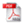Download do PDF (401 KB)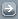Todos EventosTodos PublicaçõesTodasArtigos de Div. CientíficaArtigos em Revistas Nac.Artigos em Revistas Int.Artigos em Eventos Nac.Artigos em Eventos Int.Livros InteirosCapítulos de LivroPropriedades IntelectuaisÁudioPatentesProgramas de computadorModelos de utilidadeMarcasOutras prop. intel.TecnologiasKnow-howServiços tecnológicosTecnologias inovadorasTeses de DoutoradoDissertações de MestradoProjetos de GraduaçãoCiência e SociedadeDocumentos HistóricosMonografiasNotas de FísicaNotas TécnicasOutras Produções
 · Principal    |    CBPF    |    · Copyright 2004 - CBPF. Rio de Janeiro/BrasilCentro Brasileiro de Pesquisas Físicasv 1.1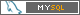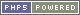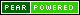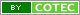Pï¿½g. gerada em 0.00 s Total de consultas SQL: 16